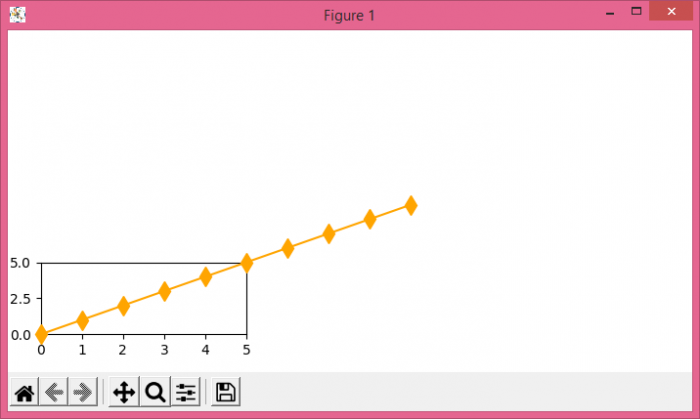# How do I let my Matplotlib plot go beyond the axes?

To let my matplotlib plot go beyond the axes, we can turn off the flag clip_on in the argument of plot() method.

## Steps

• Create xs and ys data points using numpy.

• Limit the X and Y axis range in the plot to let the line go beyond this limit, using xlim() and ylim() method.

• Plot the xs and ys data points using plot() method, where marker is a diamond shape, color is orange and clip_on=False (to go beyond the plot).

• To display the figure, use show() method.

## Example

import numpy as np
from matplotlib import pyplot as plt
plt.rcParams["figure.figsize"] = [7.00, 3.50]
plt.rcParams["figure.autolayout"] = True
xs = np.arange(10)
ys = np.arange(10)
plt.xlim(0, 5)
plt.ylim(0, 5)
plt.plot(xs, ys, marker='d', c='orange', markersize=10, clip_on=False, zorder=10)
plt.show()

## Output